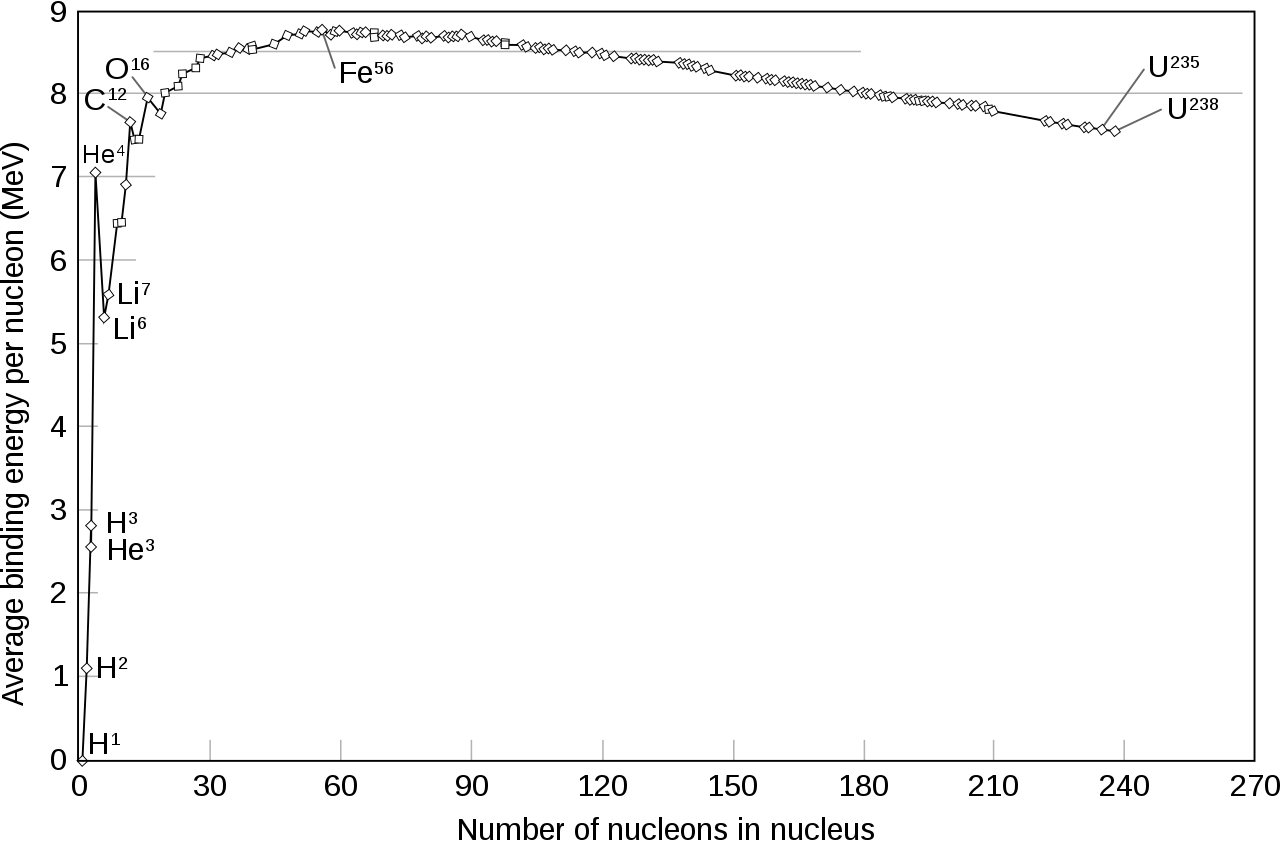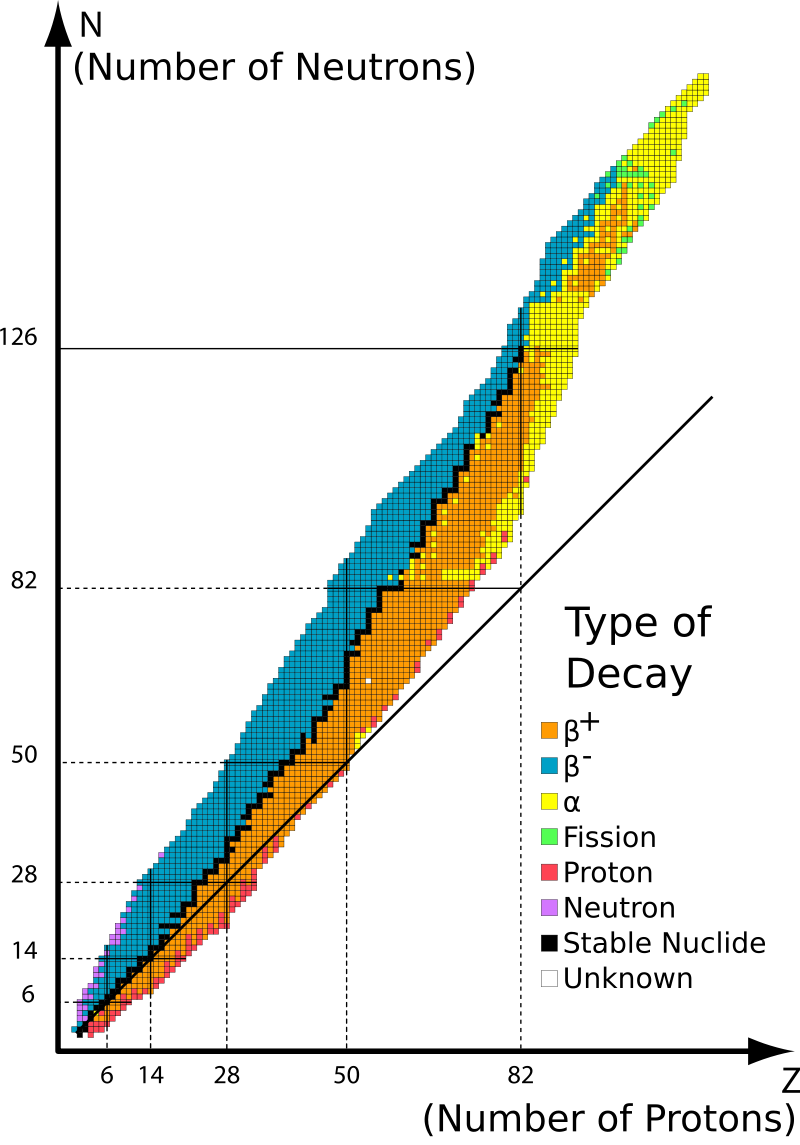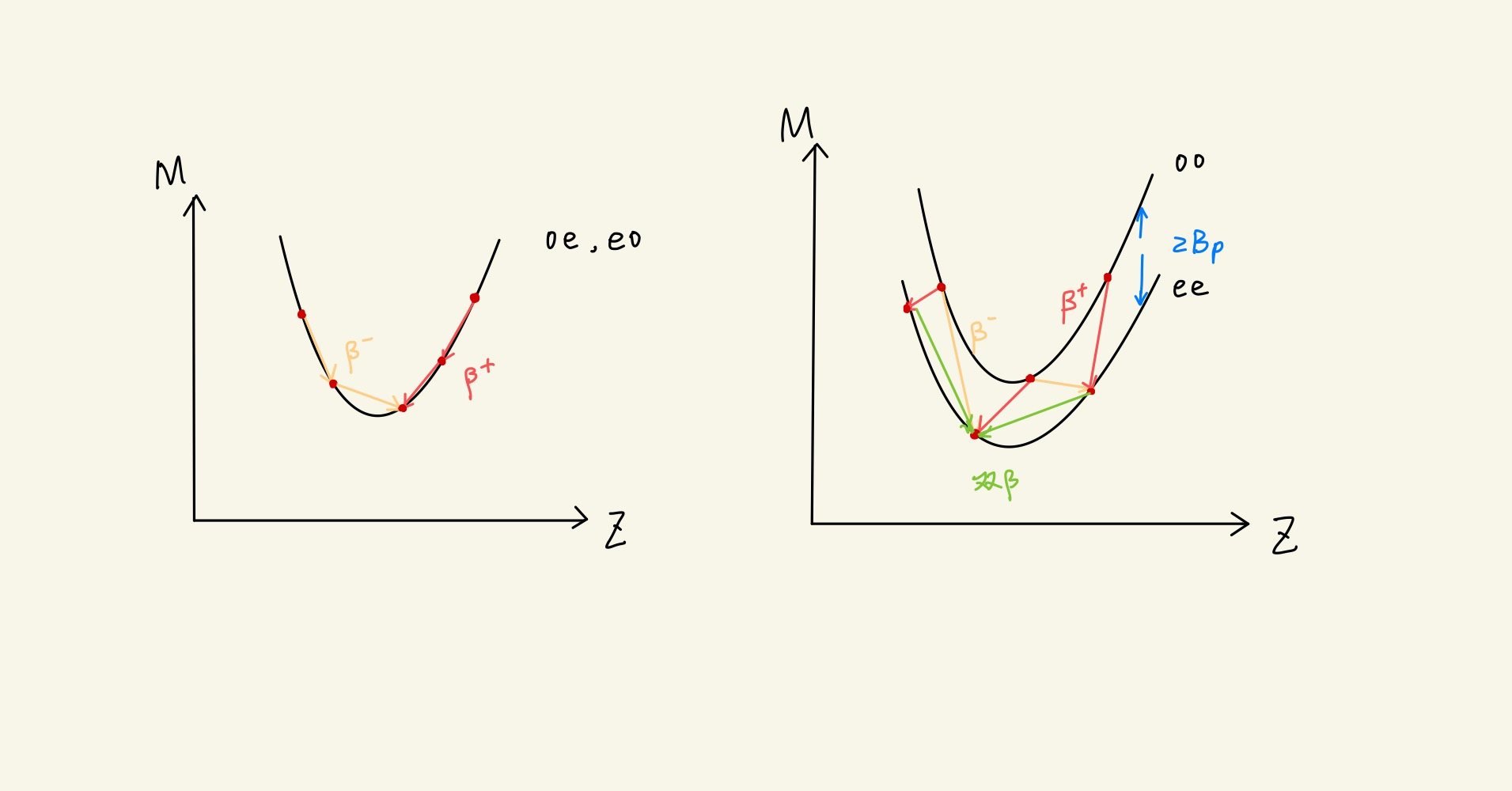## 原子核的结合能

$\Delta m(\mathrm{^4He}) = (2m_p+2m_n)-m(\mathrm{^4He})$

$M(Z,A) = m(Z,A) + Zm_e -B_e(Z)$

\begin{aligned} B(Z,A ) & = Zm_p + (A-Z)m_n -m[ \mathrm{^A_ZX} ] \\ & = (Zm_p + Zm_e) + (A-Z)m_n - (m[ \mathrm{^A_Z X}]+Zm_e)\\ & = (Zm_H + ZB_c[H]) + (A-Z)m_n-(m[ \mathrm{^A_Z X}]+Zm_e)+B_c[X,atom])\\ & \approx Zm_H + (A-Z)m_n -m[\mathrm{^A_Z X atom}] \end{aligned}

$B(Z,A) = Z\Delta_{H}+(A-Z)\Delta_n - \Delta(\mathrm{X})$

$\varepsilon = \frac{B}{A}$## 原子核稳定性的经验规律• $\beta$ 稳定线
我们将具有 $\beta$ 稳定性的核素绘制在 $N-Z$ 平面内，在 $\beta$ 稳定线以上的核素是丰中子核，会发生 $\beta^-$ 衰变； $\beta$ 稳定线以上的核素是丰中子核，会发生 $\beta^+$ 衰变或 EC。我们发现，在 $A<40$ 时，$\beta$ 稳定线近似成一条之间，且 $Z/N\approx 1$，随着质量数增加，核素中质比大于1。
$\beta$ 稳定线可以用以下经验公式表示：

$Z = \frac{A}{1.98+0.0155 A^{2/3}}$

• 核子数的奇偶性
对于稳定的核素，我们按照质子数 $Z$，中子数 $N$ 的奇偶性进行分类（odd/even）。
Z N 名称 稳定核素数目
e e 偶偶核 156
e o 偶奇核 48
o e 奇偶核 50
o o 奇奇核 5

• 重核的不稳定性
重核几乎都有 $\alpha$ 放射性。这是因为其比结合能较小，核子间结合较松。

## 原子核结合能的半经验公式

• 原子核的比结合能变化不大，由此核力应当具有饱和性。
• 核密度几乎是常量，这意味这原子核是不可压缩的。

$B = B_V + B_S + B_c$

• $B_V$体积能。与体积（质量数）成正比：

$B_V = a_VA$

• $B_S$表面能。与表面积成正比：

$B_S = a_sA^{2/3}$

• $B_c$库伦能

$B_c = -a_c Z^2A^{-1/3}$

• $B_{a}$对称能

\begin{aligned} B_{a} &= a_{a}(Z-\frac{A}{2})(N-\frac{A}{2})/A\\ & = -a_{a}(\frac{A}{2}-Z)^2A^{-1}\\ \end{aligned}

• $B_{p}$ 对能

$B_{p} = \delta a_p A^{-1/2}$

• 偶偶核：$\delta=1$
• 奇偶、偶奇核：$\delta=0$
• 奇奇核：$\delta = -1$

$B = a_VA - a_SA^{2/3}-a_cZ^2A^{-1/3}-a_{a}(\frac{A}{2}-Z)^2A^{-1}+a_p\delta A^{-1/2}$

\begin{aligned} &a_{V} = 15.835 \mathrm{MeV}\\ &a_{S} = 18.33 \mathrm{MeV}\\ &a_{c} = 0.714 \mathrm{MeV}\\ &a_{a} = 92.80 \mathrm{MeV}\\ &a_{p} = 11.2 \mathrm{MeV}\\ \end{aligned}

$m(Z,A) = Zm_p + (A-Z)m_n - B(Z,A)$

$M(Z,A) \approx ZM(\mathrm{^1H}) + (A-Z)m_n - B(Z,A)$• 奇奇核，发生 $\beta,\beta^+$ 衰变
• 奇偶核
• 发生 $\beta^+$ 衰变（$Z > Z_0$
• 发生 $\beta^-$ 衰变（$Z < Z_0$
• 偶偶核，发生 $\beta$ 衰变

同时转换两个核子

## 参考资料

1. By Table_isotopes.svg: Napy1kenobiderivative work: Sjlegg (talk) - Table_isotopes.svg, CC BY-SA 3.0, https://commons.wikimedia.org/w/index.php?curid=6703703
2. 卢希庭 原子核物理
3. 封面图 By Kjerish - Own workThis file has been extracted from another file: CNO Cycle.svg, CC BY-SA 4.0, https://commons.wikimedia.org/w/index.php?curid=54378478